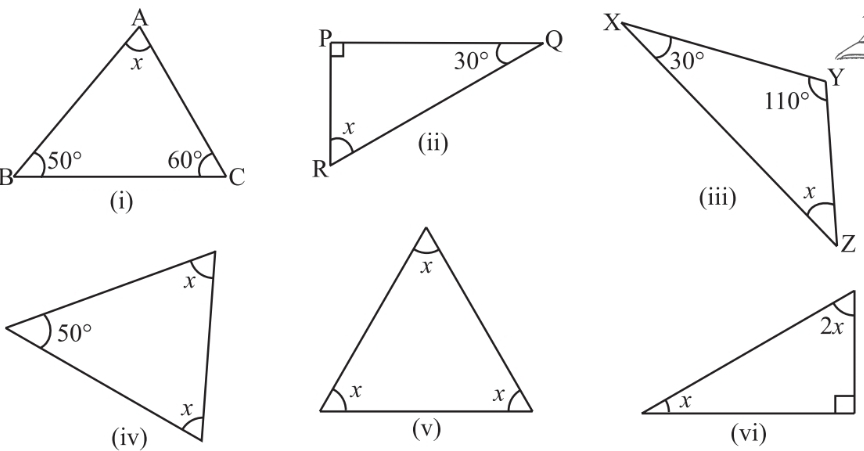#### 1.  Find the value of the unknown x in the following diagramsAs we know that the sum of the internal angles of the triangle is equal to. So,

i)ii)iii)iv)v)vi)